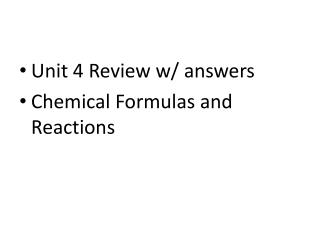DownloadDownload PresentationUnit 4 Review w/ answers Chemical Formulas and Reactions

# Unit 4 Review w/ answers Chemical Formulas and Reactions

Télécharger la présentation## Unit 4 Review w/ answers Chemical Formulas and Reactions

- - - - - - - - - - - - - - - - - - - - - - - - - - - E N D - - - - - - - - - - - - - - - - - - - - - - - - - - -
##### Presentation Transcript

1. Unit 4 Review w/ answers • Chemical Formulas and Reactions

2. Working with Equations 2Na + H2O2 2NaOH Read as: Two molecules of Na plus one molecule of H two O two yields two molecules of Na O H.

3. 2Na + H2O2 2NaOH Step 1: Circle the coefficients.

4. 2Na + H2O2 2NaOH Step 2: Put a square around the subscripts.

5. 2Na + H2O2 2NaOH Step 3: Circle the REACTANTS in RED.

6. 2Na + H2O2 2NaOH Step 4: Circle the PRODUCTS in BLUE.

7. Complete the following • For each of the following equations: • Circle the coefficients • Put a square around the subscripts • Circle the reactants in red • Circle the product/products in blue.

8. 1. 6CO2 + 6H2O C6H12O6 + 6O2

9. 2. 2 Na + Cl2 2 NaCl

10. 3. H2 + S + 2 O2 H2SO4

11. 4. H2 + Cl2 2HCl

12. 5. 2 H2 + O2 2H2O

13. 6. 2Ag + 2N +3O2 2AgNO3

14. 7. What is the coefficient of the oxygen molecule in #5? 1

15. 8. How many atoms of sulfur are there in the reactants in #3? 1

16. 9. • To balance an equation, you can only change which number? • Coefficient

17. The arrow in an equation is read as ______. means __________ YIELDS

18. In a chemical reaction, the same number of each type of atoms is present in the reactants as is found in the products. The number and type of each kind of atom cannot change; it can only be rearranged. This is known as the Law of Conservation of __________ MASS

19. In ANY chemical reaction, which of the following could be a sign that a chemical reaction has occurred? (circle all that apply) Color change Change in volume Change in shape Energy released as Change in temperature light or sound A precipitate is formed Odor change

20. In a chemical reaction, the substances you start with are the __________________. REACTANTS

21. In a chemical reaction, you substances at the end of the reaction are called ____________________ PRODUCTS

22. 15. Reactants are on the ___________ side of an equation; products are on the ____________ side of an equation. L EFT; RIGHT

23. If an equation follows the Law of Conservation of Mass, we say it is ___________________ BALANCED

24. Which equation below shows water forming when Hydrogen and oxygen gas react together? 2H2 + O2 2H2O H2O 2H2 + O2 H2O + O2 H2 Note: What does it tell you are the REACTANTS? And remember that FORMED is another word for PRODUCED or YIELDED.

25. Which of the following sets of coefficients would balance the following equation? ___ Na + __ H2O ___ NaOH + ___ H2 a. 2,1,2,1 • 2,2,2,1 • 1,2,1,2

26. When hydrogen gas is mixed with oxygen gas, does the product (water) have to be a gas? Explain why or why not. IN A CHEMICAL REACTION, A NEW SUBSTANCE IS FORMED WITH NEW PROPERTIES DIFFEENT FROM THOSE OF THE REACTANTS. HYDROGEN GAS + OXYGEN GAS = WATER, A LIQUID.

27. If 25 grams of vinegar are mixed with 10 grams of baking soda, the products (carbonic acid and carbon dioxide) will have what mass? *Hint: Law of Conservation of Mass IF YOU START WITH 35 GRAMS, YOU WILL END WITH 35 GRAMS.

28. The temperature at the beginning of a reaction, before combining the reactants, is called the ______ temperature. • Final • Initial • residual

29. A thermometer is marked so that every line is 2 degrees. If a thermometer reads -6 degrees, and the temperature drops 2 marks, the new temperature will be: a. 8 degrees b. -10 degrees c. - 2 degrees